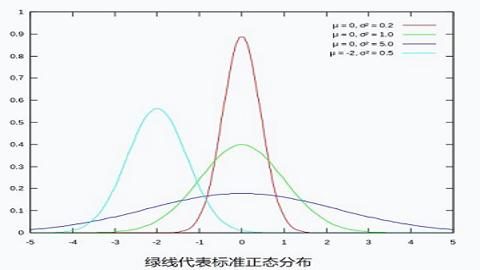# 数字图像处理/空间滤波/高斯滤波

## 效果展示## 转换函数

``````G(r) = [e^(-r²/2σ²)] / (2πσ² ^ N/2)
``````

``````G(u, v) = 1/(2πσ²) * e^[-(u² + v²)/(2σ²)]
``````

## σ 值介绍## 权重矩阵

``````import math

def get_cv(r, sigma):
return 1 / (2 * math.pi * sigma ** 2) * math.exp((-r**2) / (2 * sigma ** 2))
``````

``````[[ 0.01195525  0.02328564  0.02908025  0.02328564  0.01195525]
[ 0.02328564  0.04535423  0.05664058  0.04535423  0.02328564]
[ 0.02908025  0.05664058  0.07073553  0.05664058  0.02908025]
[ 0.02328564  0.04535423  0.05664058  0.04535423  0.02328564]
[ 0.01195525  0.02328564  0.02908025  0.02328564  0.01195525]]
``````

``````[[ 0.01441882  0.02808402  0.0350727   0.02808402  0.01441882]
[ 0.02808402  0.05470021  0.06831229  0.05470021  0.02808402]
[ 0.0350727   0.06831229  0.08531173  0.06831229  0.0350727 ]
[ 0.02808402  0.05470021  0.06831229  0.05470021  0.02808402]
[ 0.01441882  0.02808402  0.0350727   0.02808402  0.01441882]]
``````

``````[[ 10  10  10  10  10]
[ 10  10  10  10  10]
[ 10  10 200  10  10]
[ 10  10  10  10  10]
[ 10  10  10  10  10]]
``````

``````[[  0.14418818   0.28084023   0.35072701   0.28084023   0.14418818]
[  0.28084023   0.54700208   0.68312293   0.54700208   0.28084023]
[  0.35072701   0.68312293  17.06234604   0.68312293   0.35072701]
[  0.28084023   0.54700208   0.68312293   0.54700208   0.28084023]
[  0.14418818   0.28084023   0.35072701   0.28084023   0.14418818]]
``````

## 代码实现

``````import math

import numpy as np
import PIL.Image
import PIL.ImageFilter
import scipy.misc
import scipy.signal

# 概率密度函数
def get_cv(r, sigma):
return 1 / (2 * math.pi * sigma ** 2) * math.exp((-r**2) / (2 * sigma ** 2))

# 高斯滤波掩模
def get_window():
# 模糊半径为 2, sigma 为 1.5
window = np.zeros((radius * 2 + 1, radius * 2 + 1))
r = (i ** 2 + j ** 2) ** 0.5
return window / np.sum(window)

def convert_2d(r):
window = get_window()
s = scipy.signal.convolve2d(r, window, mode='same', boundary='symm')
return s.astype(np.uint8)

def convert_3d(r):
s_dsplit = []
for d in range(r.shape):
rr = r[:, :, d]
ss = convert_2d(rr)
s_dsplit.append(ss)
s = np.dstack(s_dsplit)
return s

im = PIL.Image.open('/img/jp.jpg')
im_mat = np.asarray(im)
im_converted_mat = convert_3d(im_mat)
im_converted = PIL.Image.fromarray(im_converted_mat)
im_converted.show()
``````

PIL 版本代码对应如下:

``````import PIL.Image
import PIL.ImageFilter

im = PIL.Image.open('/img/jp.jpg')